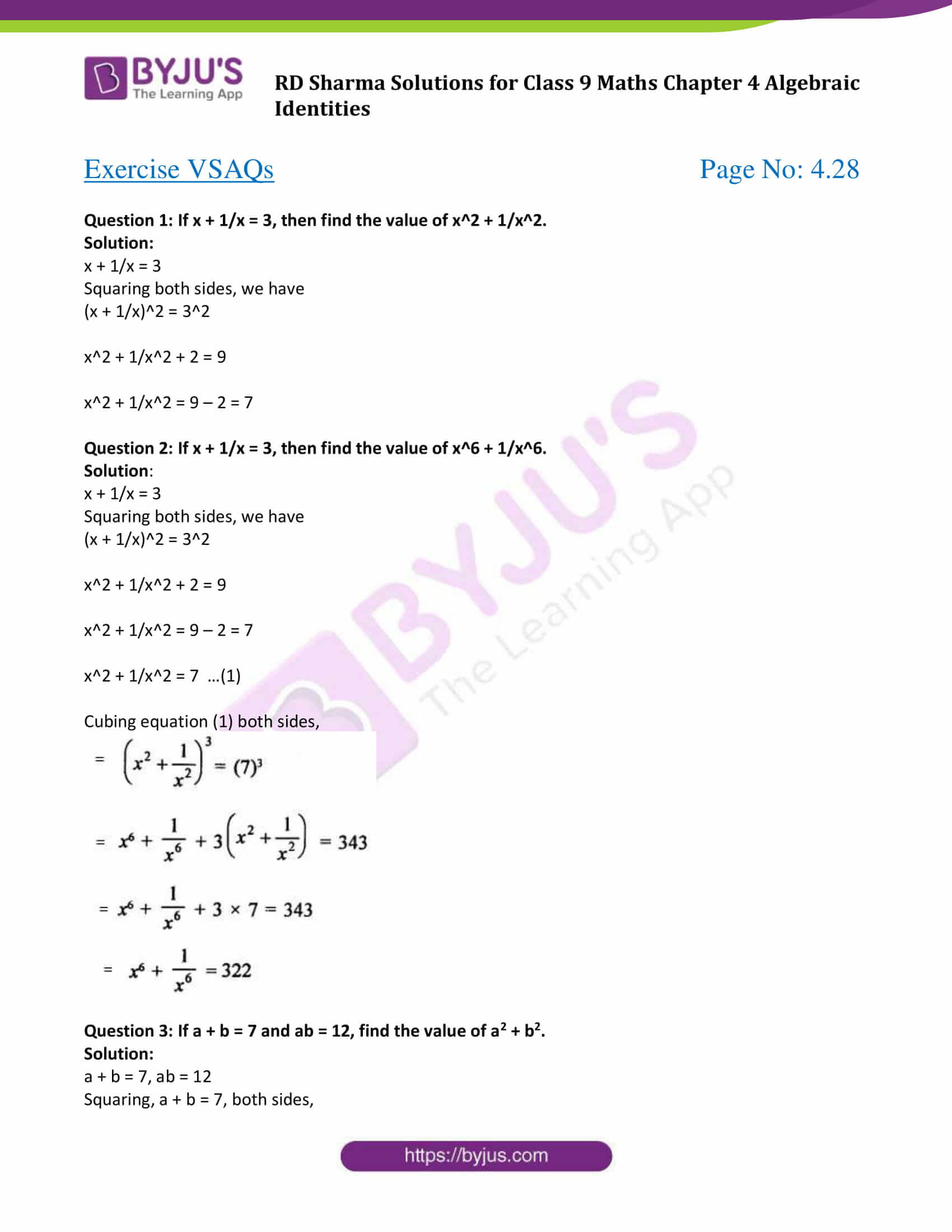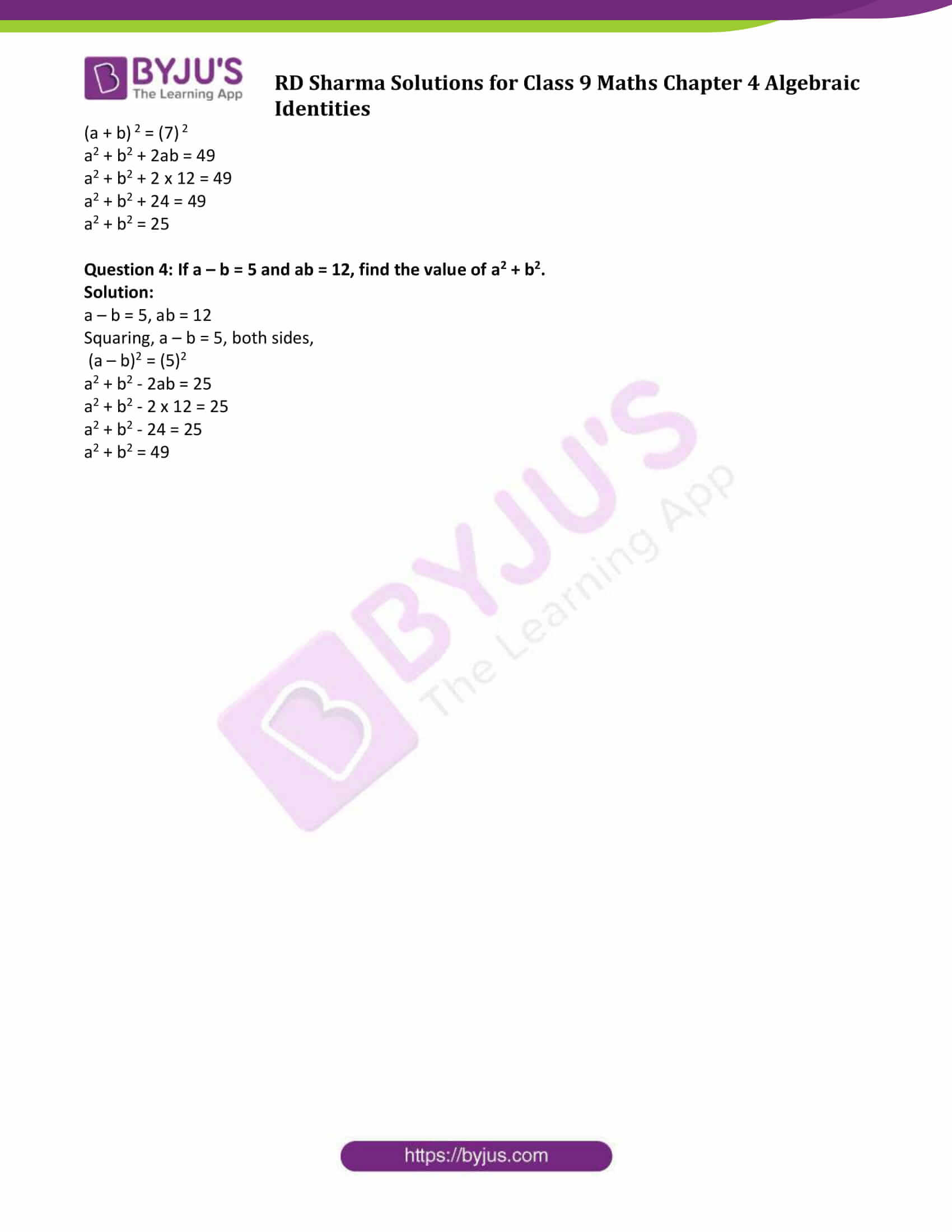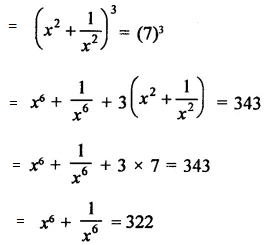# RD Sharma Solutions for Class 9 Maths Chapter 4 Algebraic Identities Exercise VSAQs

RD Sharma Class 9 Maths Chapter 4 exercise VSAQs available here. All questions are solved by BYJU’S subject experts to help students of class 9 to score good marks in exams. Students are advised to practice RD Sharma class 9 chapter 4 in order to ace the examinations without fear. Have a glimpse of class 9 Maths Chapter 4 very short type answers at below.

## Download PDF of RD Sharma Solutions for Class 9 Maths Chapter 4 Algebraic Identities Exercise VSAQs### Access Answers to Maths RD Sharma Solutions for Class 9 Chapter 4 Algebraic Identities Exercise VSAQs Page number 4.28

#### Exercise VSAQs Page No: 4.28

Question 1: If x + 1/x = 3, then find the value of x2 + 1/x2.

Solution:

x + 1/x = 3

Squaring both sides, we have

(x + 1/x)2 = 32

x2 + 1/x2 + 2 = 9

x2 + 1/x2 = 9 – 2 = 7

Question 2: If x + 1/x = 3, then find the value of x^6 + 1/x^6.

Solution:

x + 1/x = 3

Squaring both sides, we have

(x + 1/x)2 = 32

x2 + 1/x2 + 2 = 9

x2 + 1/x2 = 9 – 2 = 7

x2 + 1/x2 = 7 …(1)

Cubing equation (1) both sides,Question 3: If a + b = 7 and ab = 12, find the value of a2 + b2.

Solution:

a + b = 7, ab = 12

Squaring, a + b = 7, both sides,

(a + b) 2 = (7) 2

a2 + b2 + 2ab = 49

a2 + b2 + 2 x 12 = 49

a2 + b2 + 24 = 49

a2 + b2 = 25

Question 4: If a – b = 5 and ab = 12, find the value of a2 + b2.

Solution:

a – b = 5, ab = 12

Squaring, a – b = 5, both sides,

(a – b)2 = (5)2

a2 + b2 – 2ab = 25

a2 + b2 – 2 x 12 = 25

a2 + b2 – 24 = 25

a2 + b2 = 49

## RD Sharma Solutions for Class 9 Maths Chapter 4 Algebraic Identities Exercise VSAQs

RD Sharma Solutions Class 9 Maths Chapter 4 Algebraic Identities exercise VSAQs is based on the following topics:

• Identity for the square of a trinomial
• Identity for the cube of a Binomial
• Sum and difference of cubes
• Sum and difference of cubes Identity
• Conditional Identity